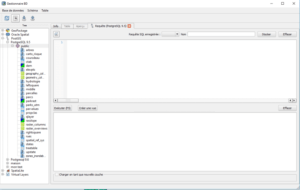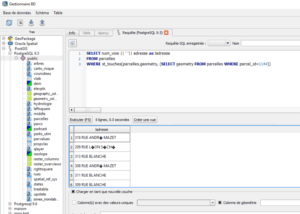# Spatial analysis with SQL: 2 -the preconceived misconceptions

In a previous article we have stated ( The analysis space with SQL: 1-Introduction ) that the most common idea is that SQL is a very good query tool on GIS tables but you must have GIS software such as ArcGIS or QGis to complete the space analysis tasks . Let’s investigate where do the misconceptions come from.

## Thedepreciationof SQL byGISsoftware

The SQL language has been a victim of the GIS software deficiencies for decades. The “flagship” GIS format between1970 and 2000, the ESRI shape file applied a reduced version of the standard SQL. It was impossible to perform sub-queries using other tables; we were confined to the fields of just one table.

To that end we must add the SQL wizards put in place by the different software , such as the ArcGis wizard:In fact, we have reduced the SQL language to, just, the WHERE clause proposing an ultra- simplistic version that was supposed to free the user from being knowledgeable with the SQL language.

During the last couple of years this situation has clearly improved. Do not quote as example anything but the QGis database manager:which allows to use all the scope of SQL, of its extensions such as Postgis, and to recover the query result and to display it in the window QGis map cartographic window .

## TheSQLlanguageiscomplicatedand notintuitive

This assertion is true … only if you do not know the basics of SQL at all!

Consider the SQL query of the final example of the previous article:

SELECT sum ( prize_terrain ) :: numeric :: money, propertyclass
FROM plots , flood zone
WHERE st_intersects ( plots.geometry, flood_zone.geometry )
GROUP BY propertyclass ;

As with all SQL queries you have two compulsory lines:

• SELECT and an information list that we wish to have as result
• FROM and a list tables list where the information is located

Then an optional line

• WHERE and the conditions to extract the outcome information

and finally one or more lines that format the outcome information (GROUP BY, ORDER BY, …)

If you take the time to answer these four questions before you start writing an SQL query, you will realize that the writing is quite intuitive:

• which is the data I want to obtain ? (-> SELECT clause)
• where is it stored ? (-> FROM clause)
• which are the conditions I want apply whether spatial or not? (-> WHERE clause)
• which format do I want for the results? (-> GROUP BY, ORDER BY …)

Let’s discuss an example: I want the addresses of all the plots bordering mine.

To build the query I ask myself the following questions:

• which is the data that I want to obtain?: I want the address of the plots (number and street) -> SELECT clause:

SELECT num_call || ‘| | address

• where is this information stored ? : in the plot table -> FROM clause:

FROM plots

• which are the conditions I want to apply , spatial or not? Just select plots that affect the plot with the plot_id = 1144-> WHERE clause:

WHERE ST_ keys (plots.geometry,   ??????)

The spatial order tests whether two geometries touch each other. In terms of plots.geometry it will browse the whole table to test whether each record touches the second part of the order .

We build this second part following the same method :

• which is the data I want get? I want the geometry of a plot -> SELECT clause:

SELECT geometry

• where is it stored ? : in the plot table -> FROM clause:

FROM plots

• which are the conditions I want to apply , spatial or not? the plot with the identifier 1144-> WHERE clause:

WHERE plot_id = 1144

We write this second part between brackets and replace the ?????? in the first part of the query

SELECT num_call || ‘| | address
FROM plots
WHERE ST_Touches (plots.geometry, (SELECT FROM geometry plots WHERE plot_id = 1144))
If we execute this request in the database manager QGis:We obtain the list of researched addresses, and if we load the result of the query in QGis we have:The plot the 1144 plot in in yellow, plots blue are those returned by the request , with their respective addresses .

If you want to be completely convinced that SQL is actually intuitive and therefore saves you time , I invite you to get the same result to the one displayed here, but by using the classic QGis tools (non sql ) or  those from ArcGis .

## What’s next?

In the next article we will discuss the PostGIS spatial operators. We will neither use the usual path. As a rule, we start with the geometries defining operators, the definition of the SRC, etc.

But similarly as when we want to learn operations with numbers, in SQL, you do not start with the transformation into imaginary numbers but with the basic operators: addition, subtraction, division, multiplication … we will discuss the small group of basic spatial functions. They are, just, around ten among the thousand available functions with Postgis. A good understanding of these functions allows you to embark, without problems, in the spatial analysis with SQL. And regarding functions on rarely used numbers (such as BIT_count or MOD or OCT), you will, only, look for, when you really have the need to.